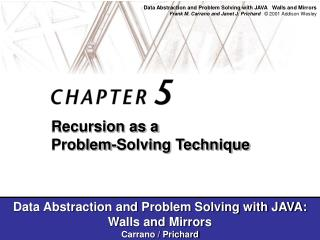DownloadDownload PresentationFigure 5.1

Figure 5.1

Télécharger la présentationFigure 5.1

- - - - - - - - - - - - - - - - - - - - - - - - - - - E N D - - - - - - - - - - - - - - - - - - - - - - - - - - -
Presentation Transcript

1. Figure 5.1 a) Five queens that cannot attack each other, but that can attack all of column 6; b) backtracking to column 5 to try another square for the queen; c) backtracking to column 4 to try another square for the queen and then considering column 5 again

2. Figure 5.2 A solution to the Eight Queens problem

3. Figure 5.3 A syntax diagram for Java identifiers

4. Figure 5.4a Trace of isId(“A2B”)

5. Figure 5.4b Trace of isId(“A2B”)

6. Figure 5.5a Trace of endPre(first, last), where strExp is +/ab-cd

7. Figure 5.5b Trace of endPre(first, last), where strExp is +/ab-cd

8. Figure 5.5c Trace of endPre(first, last), where strExp is +/ab-cd

9. Figure 5.5d Trace of endPre(first, last), where strExp is +/ab-cd